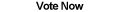Home Articles Components Code Snippets Links# C# Calculate Slope of Line

## Slope of Line

Using C#, you can write a function to calculate slope of line defined by two points. The slope of line is basically how steep a line is...

Calculating the slope is helpful for a number of C# algorithms, including image processing.

## Calculating

To calculate the slope of a line, you use the following formula:

difference in Y values / difference in X values.

You may hear it referred to as delta Y over delta X. Fittingly, a line with 0 for delta Y (meaning a horizontal line) will have a slope of 0 since it is flat.

On the other hand, a line that is perfectly vertical (meaning a delta X of 0) will have an infinite slope since it is as steep as it can get.

Due to the simplificy of the formula, the C# code will not be complicated at all. In fact, it will strongly resemble the C# distance formula function.

## C# Slope Function

The full C# slope function is in the source code that you can download at the end of the page. For the sake of accuracy, the function uses double and PointF types to calculate slope of line...

Back to C# Article List